Let's Discuss

Enquire Now

# A brief overview of Visual Question Answering (VQA)

Jul 15, 2022by, Jerin Jayaraj

Machine Learning

We know that humans can answer questions by looking at a picture or a scene. Suppose we are given a picture and a question on that image, we can surely answer it. But whether a Computer System can do that and that’s what Visual Question Answering will do. Let’s discuss more about it in the next sections.

## What is Visual Question Answering (VQA)?

Given an image and a natural language question about the image, the task is to provide an accurate natural language answer.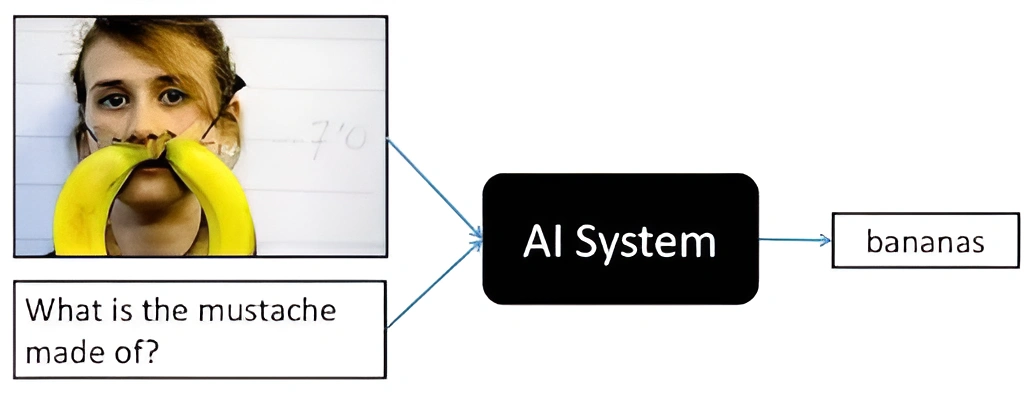From the above image, it’s pretty clear about the problem statement. On seeing an image when a question is asked , answering it is a challenging task. As a human we can answer this pretty easily but for a machine to answer it , the machine needs to learn a lot of things. So for a machine to do so, the machine needs to understand what are the objects in that image and about the question. The search and the reasoning part must be performed over the content of an image. So we need to create a model that predicts the answer of an open-ended question related to a given image. Now let’s understand the architecture.

## Basic Architecture

The VQA model comprises two Deep learning architecture CNN and RNN to accomplish this task. The CNN (Convolutional neural network) is used to obtain the image features and the RNN (Recurrent Neural network) is used to obtain the question features. These features are then combined and fed into a fully connected multi-layer perceptron (MLP) which can be then trained as a normal multi-class classifier over all the possible answer classes. The output of the network is probability distribution over all the possible answer classes.

• Extracting features from Image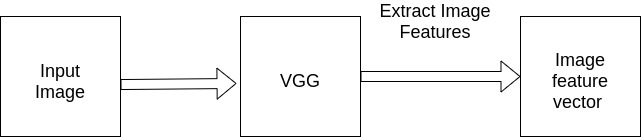The CNN used here is the VGG-19 network  to  obtain  the  image  features. VGG-19 is trained on images of size 224×224 so every image we give must be reshaped to this size. Using channel first approach and  using  RGB channel , the value of channel will be 3. So the dimension of the image will be 3x224x224. We need to expand the dimension include the number of batches.This axis dimension is required because VGG was trained on a dimension of (1,3,224,224) where first axis is for the batch size and even though we are using only one image, we have to keep the dimensions consistent. This is passed on to  VGG-19 architecture. We remove the last layer such that we get a (1,4096) dimension feature vector. Thus we obtain the image feature vector.

• Extracting features from question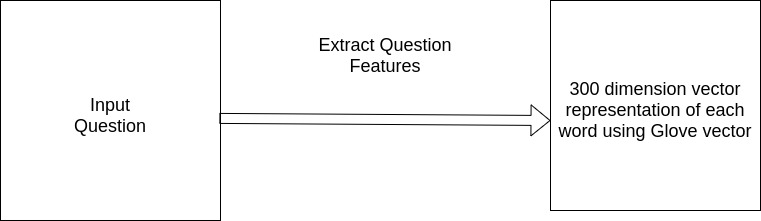To extract  question  features,  word  embedding  of  the  question  has  to  be  done. Tokenization allows the document to break down into standardized word representation eliminating punctuations. Now for each word in question, a vector is created.  The embedding is done in 300 dimension space.  Because the English language has verbs, nouns, parts of speech etc. a dimension needs to be selected which is computationally inexpensive.  Dimensions around 50 to 500 can be used.  Here, 300 is used.  The input question is mapped onto a space (maxlength, 300), where maxlength  is  the  maximum  number  of  words  allowed  in  the  question.   Glove is the unsupervised learning algorithm internally used by Spacy.  Glove reduces a token to a 300-dimensional representation.  The final vector is then returned with dimensions (1, 30, 300)

• Combined model

After extracting image features and language features from the input image and question, a sequential model is created. For the image model, image feature size is given as the input for the first layer in the sequential model and is reshaped to the required size.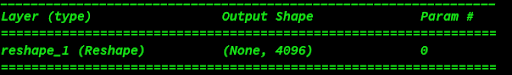Similarly for the language model, a sequential model with three LSTM layers with 512 hidden units in each is created.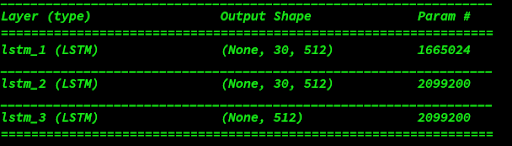The transformed image and embeddings are then fused through element-wise multiplication. This combined model is then passed into Multi Layer Perceptron (MLP) with 3 hidden layers and 1024 hidden units in each layer with tanh non-linearity and with 0.5 dropout rate to fix overfitting. Finally a dense layer with 1000 neurons and a layer with softmax activation is added to it in order to predict and select the top 1000 answers. The labels then are converted and normalized into an understandable numerical data using sklearn label encoder class.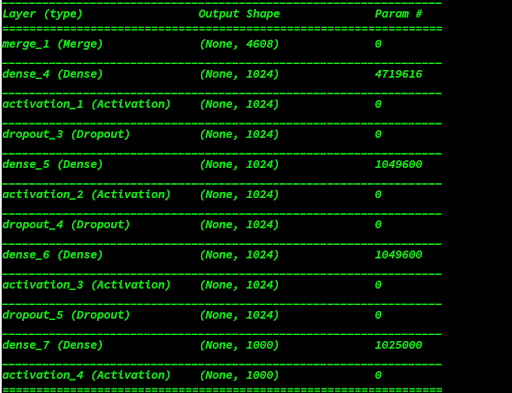#### Below is the representation of the VQA Model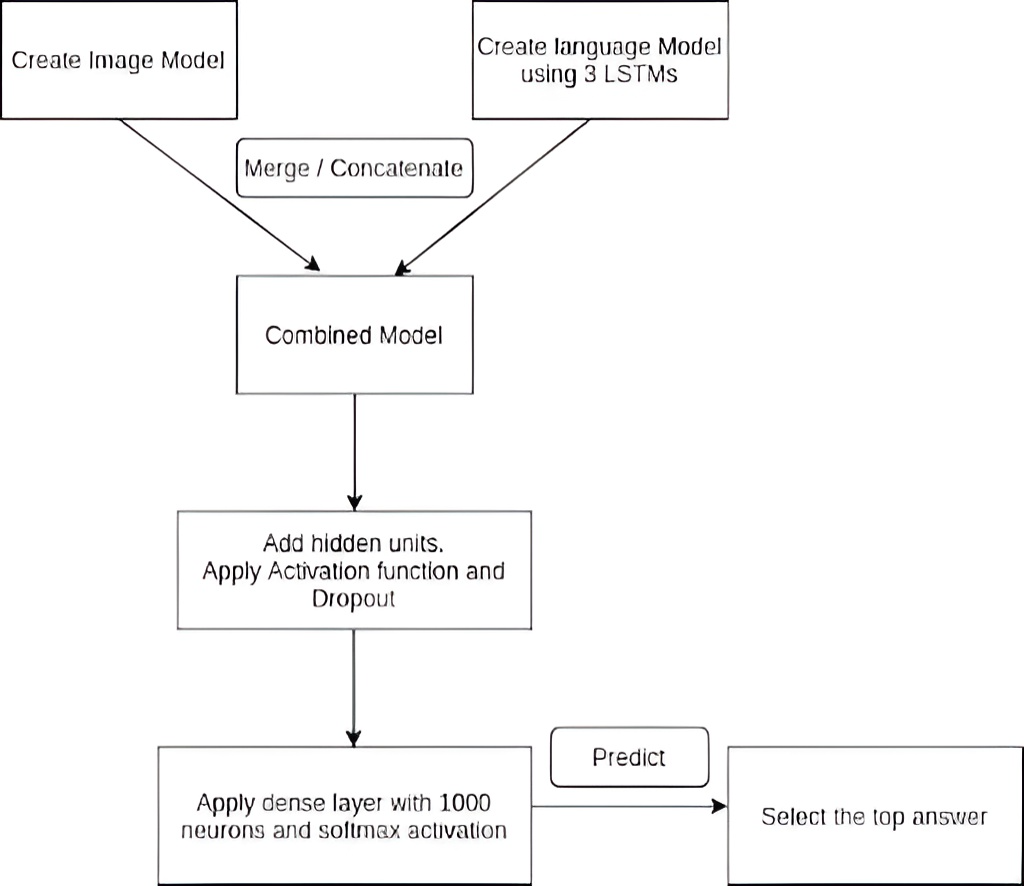## Conclusion

VQA is an algorithm that takes an image and a natural language question about the image as input and generates a natural language answer as the output.  In general, we can outline the approaches in VQA as follows:

1) Extract features from the question.

2) Extract features from the image.

3) Combine the features to generate an answer.

For text features, Long Short Term Memory (LSTM) encoders are used.

In the case of image features, CNNs pre-trained on ImageNet (VGG-19) can be used.

Regarding the generation of the answer, the approaches usually model the problem as a classification task.